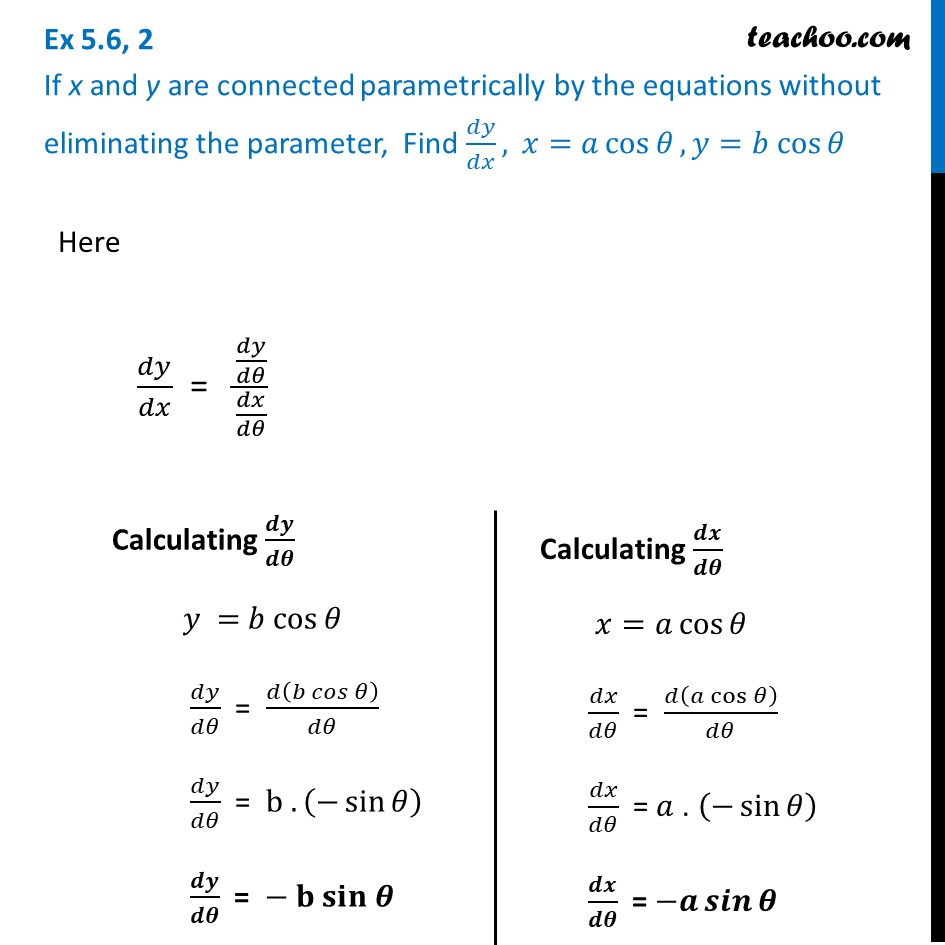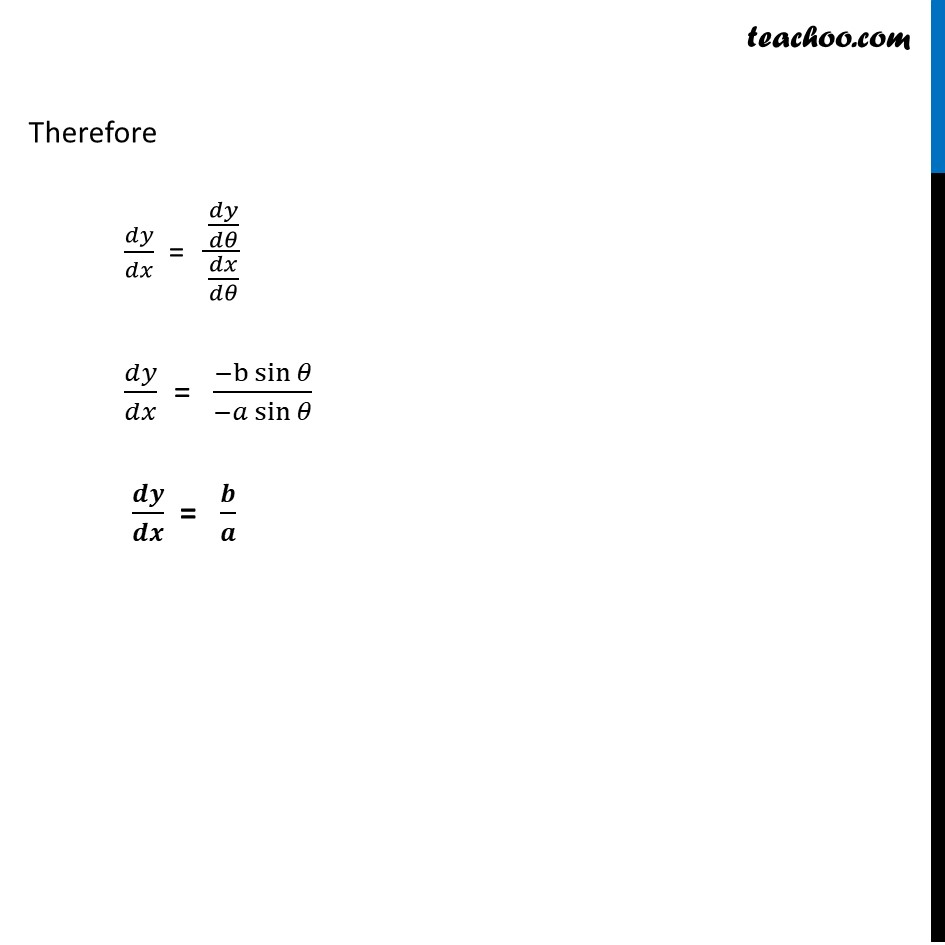Ex 5.6

Chapter 5 Class 12 Continuity and Differentiability
Serial order wiseLearn in your speed, with individual attention - Teachoo Maths 1-on-1 Class

### Transcript

Ex 5.6, 2 If x and y are connected parametrically by the equations without eliminating the parameter, Find 𝑑𝑦/𝑑𝑥, 𝑥=𝑎 cos⁡𝜃, 𝑦=𝑏 cos⁡𝜃Here 𝑑𝑦/𝑑𝑥 = (𝑑𝑦/𝑑𝜃)/(𝑑𝑥/𝑑𝜃) Calculating 𝒅𝒚/𝒅𝜽 𝑦 = 𝑏 cos⁡𝜃 𝑑𝑦/𝑑𝜃 = 𝑑(𝑏 𝑐𝑜𝑠⁡𝜃)/𝑑𝜃 𝑑𝑦/𝑑𝜃 = b . (−sin⁡𝜃 ) 𝒅𝒚/𝒅𝜽 = − 𝐛 𝐬𝐢𝐧 𝜽 Calculating 𝒅𝒙/𝒅𝜽 𝑥=𝑎 cos⁡𝜃 𝑑𝑥/𝑑𝜃 = 𝑑(𝑎 cos⁡𝜃 )/𝑑𝜃 𝑑𝑥/𝑑𝜃 = 𝑎 . (−sin⁡𝜃 ) 𝒅𝒙/𝒅𝜽 = −𝒂 𝒔𝒊𝒏⁡𝜽